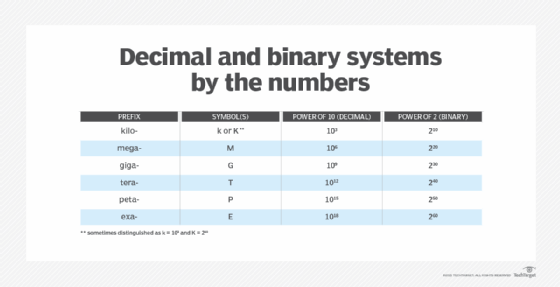# What is a gigabit in data communications? – TechTarget Definition

Date:

### What is a gigabit (Gb)?

In data communications, a gigabit (Gb) is 1 billion bits, or 1,000,000,000 (that is, 109) bits. It’s commonly used for measuring the amount of data that is transferred in a second between two telecommunication points. For example, Gigabit Ethernet (GbE) is a high-speed form of Ethernet, a local area network technology that can deliver data transfer rates of as fast as 400 Gb per second (Gbps).

Some sources define a gigabit as 1,073,741,824 bits (about 230). Although the bit is a unit of the binary number system, bits in data communications are discrete signal pulses and have historically been counted using the decimal number system.

However, there is a fair amount of confusion over bit prefix multipliers and the differences between the decimal and binary number systems in general, leaving many uncertain about whether a gigabit is equal to 1 billion bits or closer to 1.074 billion bits.

### Gigabit in binary vs. decimal numbering systems

The smallest unit of data in computing is the bit, which has a value of either 1 or 0. This, in itself, is well established and understood. In fact, it is what the binary number system is based on. The problem arises when multipliers are used to quantify the bits and different number systems are applied to those values.

The decimal system uses base-10 calculations when assigning multipliers to bits. Base-10 calculations are fairly straightforward because they follow the same system that we use in our everyday lives. For example, 102 is 100; 103 is 1,000; 104 is 10,000; and so on. When we pay our electric bills, we are using a base-10 number system. Such a system easily translates to the prefix multipliers often used for data communications:

• 1 kilobit (Kb) = 103 bits
• 1 megabit (Mb) = 106 bits
• 1 gigabit (Gb) = 109 bits
• 1 terabit (Tb) = 1012 bits
• 1 petabit (Pb) = 1015 bits
• 1 exabit (Eb) = 1018 bits

In this system, 1 Gb equals 109 bits, which comes to 1 billion bits. However, some sources use the binary system instead of the decimal system when referring to data rates but continue to use the same prefix name to describe those values. Unlike the decimal system, the binary system uses base-2 calculations when assigning values, which is why gigabit is sometimes defined as being closer to 1.074 billion bits, or 230 bits.Table illustrating the differences in quantities between the decimal and binary systems for common prefixes

Because of this confusion, the industry has been steadily moving toward a set of prefix multipliers specific to the base-2 standard. For example, the following multipliers are the binary counterparts to the decimal multipliers listed above:

• 1 kibibit (Kib) = 210 bits
• 1 mebibit (Mib) = 220 bits
• 1 gibibit (Gib) = 230 bits
• 1 tebibit (Tib) = 240 bits
• 1 pebibit (Pib) = 250 bits
• 1 exbibit (Eib) = 260 bits

The base-2 standard is also being adopted for byte-related multipliers. For example, 1 gigabyte (GB) equals 1 billion bytes (109), and 1 gibibyte (GiB) equals 1,073,741,824 bytes (again, about 230).Table illustrating the differences between measuring in binary vs. decimal systems

Despite the movement toward base-2 multipliers, such as gibibit, many vendors and other sources continue to use base-10 multiplier names with base-2 calculations. For this reason, people evaluating network and communication systems should be certain that they understand how the terminology is being used so they can properly assess and compare systems.

### Gigabit vs. gigabyte

Gigabit is often contrasted or confused with gigabyte, which is generally used to describe data storage — the amount of data being stored in a device or the capacity of the device storing the data. Byte-related prefixes are often used because the byte is the smallest addressable unit of memory in many computer architectures. A byte contains 8 bits, but those bits are processed as a single unit or combined with other bytes to create larger units.

Although byte-related multipliers are generally used for data at rest, they sometimes refer to data transfers or to storage bandwidth or throughput. This too can cause confusion when evaluating or comparing systems or services, so those evaluating these systems must be sure they understand the metrics being used in this area as well.Diagram illustrating the differences between data at rest vs. data in motion vs. data in use

Share post:

Popular

### Apple marks World AIDS Day with (RED)

December 1, 2023 UPDATE Apple marks World AIDS...

### AI’s Impact On Venture Capital And 5 Tips For Success

AI stands as the bedrock for innovative products...

### WhatsApp now lets you use secret codes to lock your private chats

WhatsApp is making its Chat Lock tool even...

### TikTok: US judge blocks Montana’s ban citing free speech

Chinese-owned app welcomes the ruling against "unconstitutional law"...